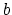Next: Introduction Up: NAMD 2.9 User's Guide Previous: Contents   Contents   Index

## List of Figures

1. Graph of van der Waals potential with and without switching
2. Graph of electrostatic potential with and without shifting function
3. Graph of electrostatic split between short and long range forces
4. Example of cutoff and pairlist distance uses
5. Graph showing a slice of a ramp potential, showing the effect of mgridforcevoff
6. Example of a collective variables (colvar) configuration. The colvar'' is defined as the difference between two distances, each calculated between the centers of mass of two atom groups. The second colvar'' holds the coordination number (i.e. the number of contacts) within a radius of 6 Å between two groups. The third colvar alpha'' measures the degree of-helicity of the protein segment between residues 1 and 10. A moving harmonic restraint is applied to the colvars'' and'', each rescaled by means of width parametersand; the centers of the restraint,and, evolve with the simulation time. The joint histogram of alpha'' and'' is also recorded on-the-fly.
7. Dual topology description for an alchemical simulation. Case example of the mutation of alanine into serine. The lighter color denotes the non-interacting, alternate state.
8. Convergence of an FEP calculation. If the ensembles representative of statesandare too disparate, equation (56) will not converge (a). If, in sharp contrast, the configurations of stateform a subset of the ensemble of configurations characteristic of state, the simulation is expected to converge (b). The difficulties reflected in case (a) may be alleviated by the introduction of mutually overlapping intermediate states that connectto(c). It should be mentioned that in practice, the kinetic contribution,, is assumed to be identical for stateand state.
9. Relationship of user-definedto coupling of electrostatic or vdW interactions to a simulation, given specific values of alchElecLambdaStart or alchVdwLambdaEnd.
10. Sample TI data (against). The blue shaded area shows the integral with fine sampling close to the end point. The red area shows the difference whenvalues are more sparse. In this example, insufficient sampling before0.1 can result in a large overestimation of the integral. Beyond0.2, sparser sampling is justified as dE/dis not changing quickly.
11. Schematics of the aMD method. When the original potential (thick line) falls below a threshold energy(dashed line), a boost potential is added. The modified energy profiles (thin lines) have smaller barriers separating adjacent energy basins.

http://www.ks.uiuc.edu/Research/namd/